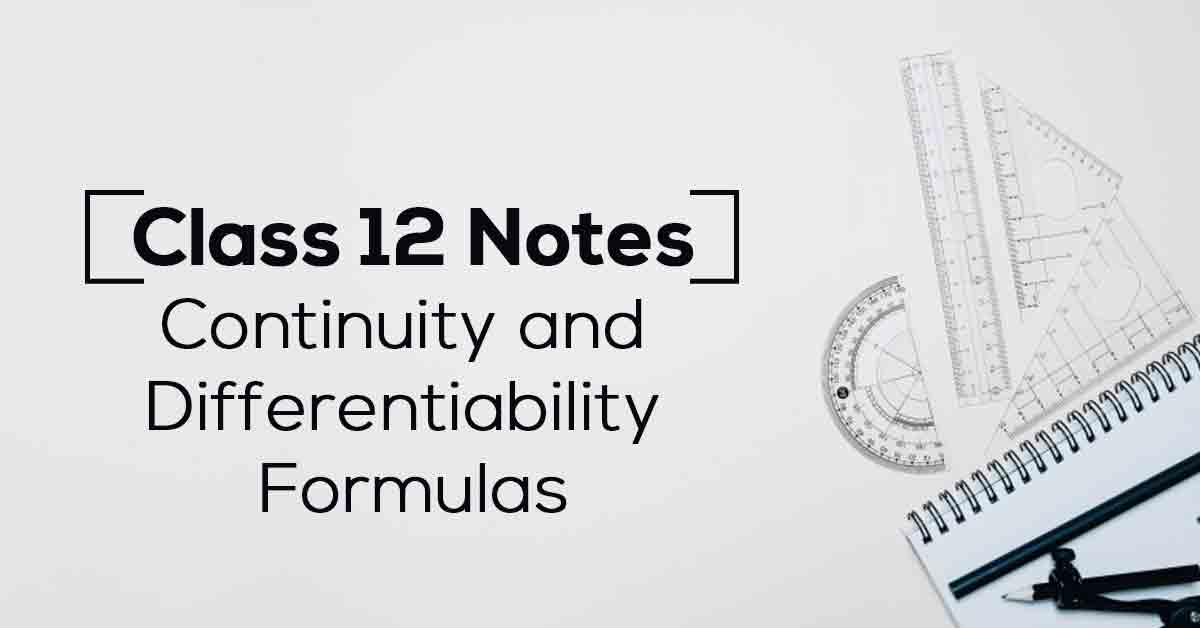Continuity and Differentiability Class 12 Notes | Vidyakul
×

# Continuity and Differentiability Class 12 Notes## Class 12 Maths Chapter 5 Continuity and Differentiability Class 12 Notes – PDF Download

Limits, Continuity, and Differentiability can, in fact, be termed as the building blocks of Calculus as they form the basis of entire Calculus. The basic concept of limit of a function lays the groundwork for the concepts of continuity and differentiability. These concepts can, in fact, be called the natural extensions of the concept. Know More about these in Continuity and Differentiability Class 12 Notes List.

The topics and sub-topics covered in Continuity and Differentiability Class 12 Notes are:

5.1 Introduction

5.2 Continuity

5.2.1 Algebra of continuous functions

5.3. Differentiability

5.3.1 Derivatives of composite functions

5.3.2 Derivatives of implicit functions

5.3.3 Derivatives of inverse trigonometric functions

5.4 Exponential and Logarithmic Functions

5.5. Logarithmic Differentiation

5.6 Derivatives of Functions in Parametric Forms

5.7 Second Order Derivative

5.8 Mean Value Theorem.

Download the FREE PDF of Continuity and Differentiability Class 12 Notes and start your preparation with Vidyakul!